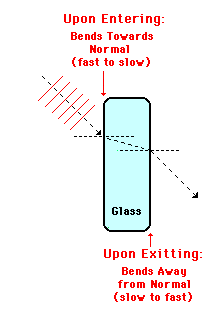Does refraction change the direction of light when it passes from air to glass?

As long as the light strikes the glass with an angle of incidence greater than ${0}^{o}$, then yes, the direction will change. This occurs anytime you have 2 materials with different indices of refraction.The angle of refraction can be calculated using Snell's Law (${n}_{1} \sin {\Theta}_{1} = {n}_{2} \sin {\Theta}_{2}$), where ${\Theta}_{1}$ is the angle of incidence and you know the two indices of refraction.